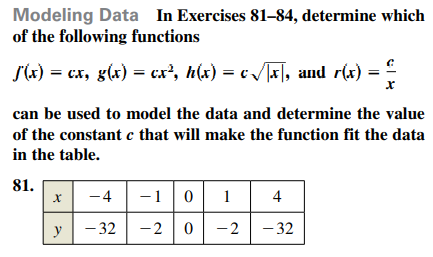### Still have math questions?

Algebra
QuestionModeling Data In Exercises , determine which of the following functions $$J ( x ) = c x , g ( x ) = c x ^ { 2 } , h ( x ) = c \sqrt { | x | }$$ , and $$r ( x ) = \frac { c } { x }$$

can be used to model the data and determine the value of the constant $$c$$ that will make the function fit the data in the table.